# Vector product

(diff) ← Older revision | Latest revision (diff) | Newer revision → (diff)

of a vector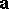by a vector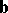in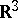The vector, denoted by the symbol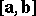or, satisfying the following requirements:

1) the length ofis equal to the product of the lengths of the vectorsandby the sine of the anglebetween them, i.e.2)is orthogonal to bothand;

3) the orientation of the vector triple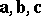is the same as that of the (standard) triple of basis vectors. See Vector algebra.

If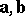have coordinatesand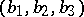with respect to an orthonormal basis in, then the coordinates of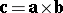are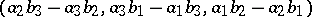.